GSAK (Geocaching Swiss Army Knife)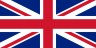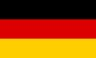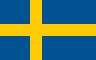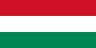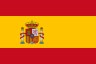Contents - Index

RegExData (function)

RegExData(sExpression, sData, nNumber) : String

Use this function to return the matched data instance of sExpression found in sData. Use with RegExCount to iterate through all the matches in a regular expression.

sExpression = The regular expression used for this search
sData = The data to search
nNumber = reference number of the matched regular expression. If zero, then this function will return all matches as one long string.

Sample 1

 # pull out all numbers in a string \$data = "My 3 dogs have 432 fleas and 25 ticks" \$expression = "\d*" \$count = RegExCount(\$expression,\$data) \$x = 0 While \$count > \$x   \$x = \$x + 1   \$found = RegExData(\$expression,\$data,\$x)   MsgOk Msg=\$found EndWhile

This code will show 3 messages. The messages will show 3, 432, and 25 respectively.

Sample 2

 \$data = "My 3 dogs have 432 fleas and 25 ticks" \$expression = "\d*" \$MatchAll = RegExData(\$expression,\$data,0) msgOk msg=\$MatchAll

This code will show only one message: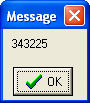Note: the regular expression engine has been set to ignore case .

Alpha List         Category List